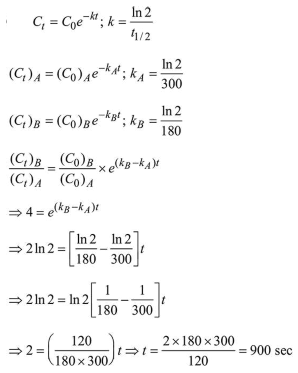# A flask contains a mixture of compounds A and B. Both compounds decompose by first-order kinetics.

Question:

A flask contains a mixture of compounds A and B. Both compounds decompose by first-order kinetics. The halflives for $\mathrm{A}$ and $\mathrm{B}$ are $300 \mathrm{~s}$ and $180 \mathrm{~s}$, respectively. If the concentrations of $A$ and $B$ are equal initially, the time required for the concentration of $A$ to be four times that of $\mathrm{B}$ (in $\mathrm{s}$ ) is: (Use $\ln 2=0.693$ )

1. 180

2. 900

3. 300

4. 120

Correct Option: , 2

Solution: